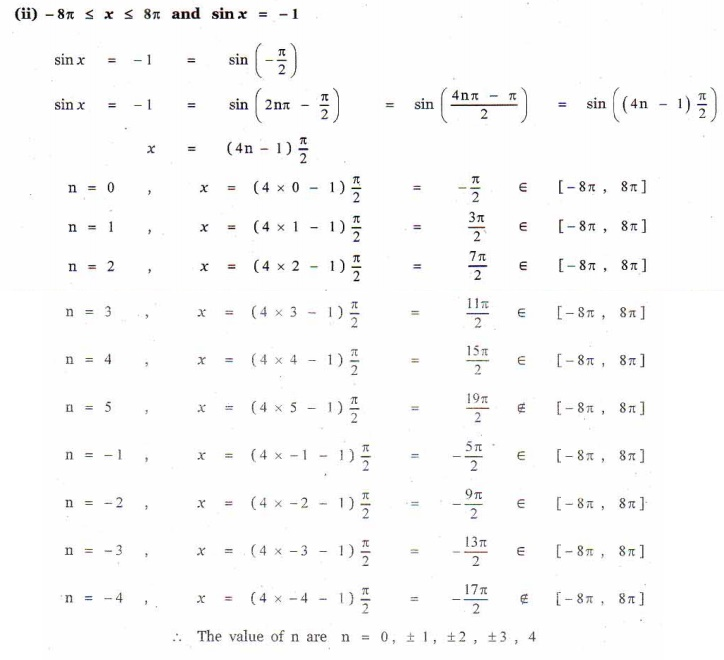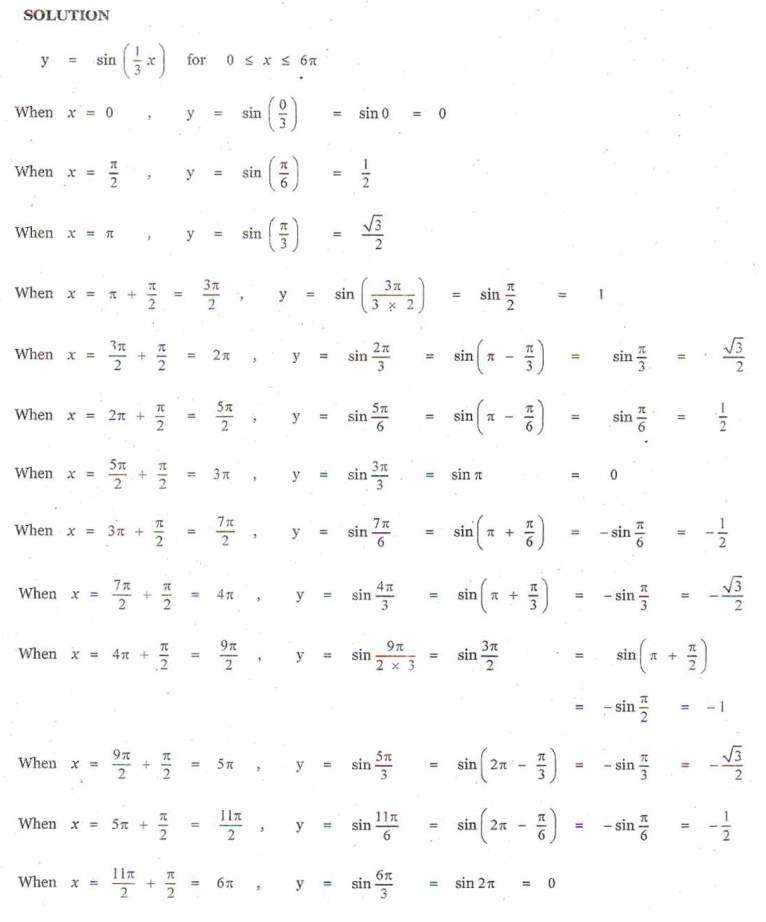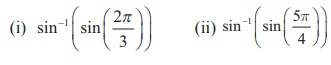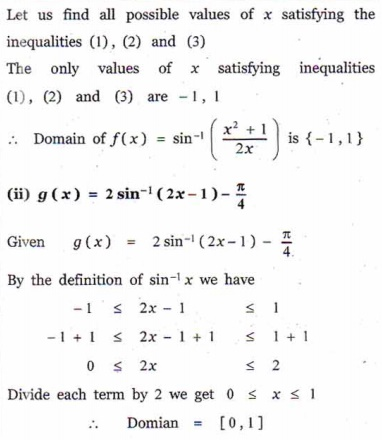Home | | Maths 12th Std | Exercise 4.1: Sine Function and Inverse Sine Function

# Exercise 4.1: Sine Function and Inverse Sine Function

Maths Book back answers and solution for Exercise questions - Mathematics : Inverse Trigonometric Functions: Sine Function and Inverse Sine Function: Exercise Questions with Answer, Solution

EXERCISE 4.1

1. Find all the values of such that

(i) -10π ≤ x ≤ 10π and sin x = 0

(ii) -8π ≤ x ≤ 8π and sin x = -1.2. Find the period and amplitude of

(i) y = sin7x

(ii) y = -sin ( 1/3 x )

(iii) y = 4 sin(-2x) .3. Sketch the graph of = sin ([1/3] x) for 0 ≤ < 6π .4.Find the value of5. For what value of does sin = sin?6. Find the domain of the following7. Find the value of sin1